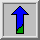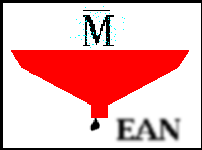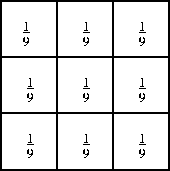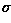# Mean FilterCommon Names: Mean filtering, Smoothing, Averaging, Box filtering

## Brief Description

Mean filtering is a simple, intuitive and easy to implement method ofsmoothing images, i.e. reducing the amount of intensity variation between one pixel and the next. It is often used toreduce noise in images.## How It Works

The idea of mean filtering is simply to replace each pixel value in an image with the mean (`average') value of its neighbors, including itself. This has the effect of eliminating pixel values which are unrepresentative of their surroundings. Mean filtering is usually thought of as aconvolution filter. Like other convolutions it is based around a kernel, which represents the shape and size of the neighborhood to be sampled when calculating the mean. Often a 3×3 square kernel is used, as shown in Figure 1, although larger kernels (e.g. 5×5 squares) can be used for more severe smoothing. (Note that a small kernel can be applied more than once in order to produce a similar but not identical effect as a single pass with a large kernel.)Figure 1 3×3 averaging kernel often used in mean filtering

Computing the straightforward convolution of an image with this kernel carries out the mean filtering process.## Guidelines for Use

Mean filtering is most commonly used as a simple method for reducing noise in an image.

We illustrate the filter usingThe imageshows the original corrupted by Gaussian noise with a mean of zero and a standard deviation () of 8.

The imageshows the effect of applying a 3×3 mean filter. Note that the noise is less apparent, but the image has been `softened'. If we increase the size of the mean filter to 5×5, we obtain an image with less noise and less high frequency detail, as shown inThe same image more severely corrupted by Gaussian noise (with a mean of zero and aof 13) is shown inThe imageis the result of mean filtering with a 3×3 kernel.

An even more challenging task is provided by. It shows an image containing `salt and pepper' shot noise.

The imageshows the effect of smoothing the noisy image with a 3×3 mean filter. Since the shot noise pixel values are often very different from the surrounding values, they tend to significantly distort the pixel average calculated by the mean filter.

Using a 5×5 filter instead givesThis result is not a significant improvement in noise reduction and, furthermore, the image is now very blurred.

These examples illustrate the two main problems with mean filtering, which are:

• A single pixel with a very unrepresentative value can significantly affect the mean value of all the pixels in its neighborhood.

• When the filter neighborhood straddles an edge, the filter will interpolate new values for pixels on the edge and so will blur that edge. This may be a problem if sharp edges are required in the output.

Both of these problems are tackled by the median filter, which is often a better filter for reducing noise than the mean filter, but it takes longer to compute.

In general the mean filter acts as alowpass frequency filter and, therefore, reduces the spatial intensity derivatives present in the image. We have already seen this effect as a `softening' of the facial features in the above example. Now consider the imagewhich depicts a scene containing a wider range of different spatial frequencies. After smoothing once with a 3×3 mean filter we obtainNotice that the low spatial frequency information in the background has not been affected significantly by filtering, but the (once crisp) edges of the foreground subject have been appreciably smoothed. After filtering with a 7×7 filter, we obtain an even more dramatic illustration of this phenomenon inCompare this result to that obtained by passing a 3×3 filter over the original image three times in## Common Variants

Variations on the mean smoothing filter discussed here includeThreshold Averaging wherein smoothing is applied subject to the condition that the center pixel value is changed only if the difference between its original value and the average value is greater than a preset threshold. This has the effect that noise is smoothed with a less dramatic loss in image detail.

Other convolution filters that do not calculate the mean of a neighborhood are also often used for smoothing. One of the most common of these is the Gaussian smoothing filter.

## Interactive Experimentation

You can interactively experiment with this operator by clicking here.

## Exercises

1. The mean filter is computed using a convolution. Can you think of any ways in which the special properties of the mean filter kernel can be used to speed up the convolution? What is the computational complexity of this faster convolution?

2. Use an edge detector on the imageand note the strength of the output. Then apply a 3×3 mean filter to the original image and run the edge detector again. Comment on the difference. What happens if a 5×5 or a 7×7 filter is used?

3. Applying a 3×3 mean filter twice does not produce quite the same result as applying a 5×5 mean filter once. However, a 5×5 convolution kernel can be constructed which is equivalent. What does this kernel look like?

4. Create a 7×7 convolution kernel which has an equivalent effect to three passes with a 3×3 mean filter.

5. How do you think the mean filter would cope with Gaussian noise which was not symmetric about zero? Try some examples.

## References

R. Boyle and R. Thomas Computer Vision: A First Course, Blackwell Scientific Publications, 1988, pp 32 - 34.

E. Davies Machine Vision: Theory, Algorithms and Practicalities, Academic Press, 1990, Chap. 3.

D. Vernon Machine Vision, Prentice-Hall, 1991, Chap. 4.

## Local Information